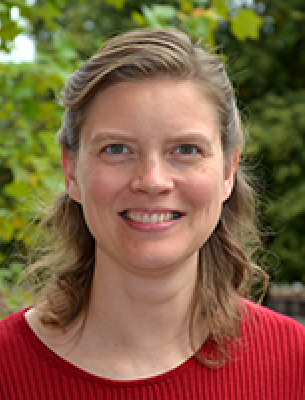# Amy Wehe

PROFESSOR
Mathematics School of Health and Natural SciencesOffice: Edgerly Hall 301D
Office Hours
Fall 2023

Monday and Wednesday 11am - 12pm
Tuesday 9:45am - 10:45am

## Courses Taught

Introduction to Functions (MATH 1250)
Precalculus (MATH 1300)
Applied Statistics (MATH 1700)
Discrete Mathematics (MATH 1900)
Informal Geometry (MATH 2000)
Calculus I (MATH 2300)
Calculus II (MATH 2400)
Linear Algebra (MATH 2600)
Geometry (MATH 3000)
History of Mathematics (MATH 3200)
Calculus IV (MATH 4150)
Probability and Statistics I (MATH 4200)
Probability and Statistics II (MATH 4250)
Abstract Algebra (MATH 4300)

## Background

Ph.D., Iowa State University. 2005.

Combinatorial Matrix Theory
Spectral Graph Theory
"Teaching and Learning"

Ph.D., Iowa State University. 2005.

Ph.D., Iowa State University. 2005.